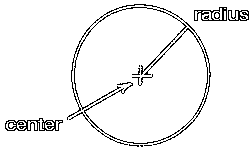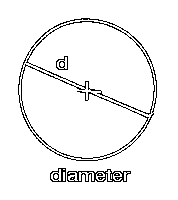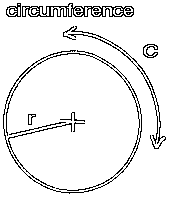# The CircleThe circle is a set of points equally distant from one central point. This central point is called the center of the circle. Here the center is shown with a small cross.

The distance from the center to the curve of the circle is called the radius. Here the radius is shown as a line from the center to the curve of the circle.The distance across the circle is called the diameter. The diameter always passes through the center of the circle. The diameter is equal in length to twice the radius.The distance all the way around the circle is called the circumference. The circumference is usually symbolized with a 'C' as shown here. The length of the circumference is equal to 2 times pi times the radius.

Usually, as here, the radius is symbolized with an 'r'.

Custom Search